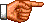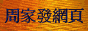# 點算的奧秘：普通母函數

「母函數」(亦作「生成函數」Generating Function)是在「點算組合學」上有廣泛應用的工具，「 點算組合學」的幾乎每個領域都有相應的「母函數」，本文只擬介紹與前面介紹過的幾種點算問題(排列組合問 題、集合劃分問題、整數分割問題、分配問題)密切相關的「母函數」。

1 + 3x + 3x2 + x3

C(n, 0) + C(n, 1)x + C(n,2)x2 + ... + C(n, n)xn    (1)

Σ0 ≤ r ≤ nC(n, r)xr    (2)

(1 + x)n    (3)

Σ0 ≤ r < ∞ arxr = a0 + a1x + a2x2 + ...    (4)

 (1 + x)3 = (1 + x) × (1 + x) × (1 + x) = 1 × 1 × 1 + 1 × 1 × x + 1 × x × 1 + 1 × x × x + x × 1 × 1 + x × 1 × x + x × x × 1 + x × x × x = 1 + 3x + 3x2 + x3

(1 + x) × (x + x2 + x3 + x4 + x5 + x6 + x7) × (x + x2)

Σ0 ≤ r < ∞ xr = 1 + x + x2 + x3 + ... = 1 / (1 − x)    (5)

Σ0 ≤ r < k xr = 1 + x + x2 + ... xk = (1 − xk+1) / (1 − x)    (6)

(1 ± x)n = [(±x) + 1]n = Σ 0 ≤ r ≤ n C(n, r) (±x)r    (7)

(1 ± x)−n = [(±x) + 1]−n = Σ 0 ≤ r < ∞ C(−n, r) (±x)r    (8)

 C(−n, r) = (−1)r n(n + 1)(n + 2) ... (n + r − 1) / r! = (−1)r (n + r − 1)! / [(n − 1)! × r!] = (−1)r C(n + r − 1, r)

 (1 ± x)−n = Σ 0 ≤ r < ∞ (−1)r C(n + r − 1, r) (±x)r = Σ 0 ≤ r < ∞ C(n + r − 1, r) (−1 × ±x)r    (9)

(1 − x)−n = Σ 0 ≤ r < ∞ C(n + r − 1, r) xr    (10)

 (x6 + x7 + x8 + x9 + x10)3 = [x6(1 + x + x2 + x3 + x4)]3 = x18(1 + x + x2 + x3 + x4)3

 x18[(1 − x5) / (1 − x)]3 = x18(1 − x5)3(1 − x)−3

x18 × Σ0 ≤ r ≤ 3 C(3, r) (−1)r x5r × Σ0 ≤ s < ∞ C(2 + s, s) xs

 C(3, 0) × (−1)0 × C(2 + 10, 10) + C(3, 1) × (−1)1 × C(2 + 5, 5) + C(3, 2) × (−1)2 × C(2 + 0, 0) = 1 × 66 − 3 × 21 + 3 × 1 = 6 □

 (x + x2 + x3 + ...) (x4 + x8 + x12 + ...) (x5 + x10 + x15 + ...) = x10 (1 + x + x2 + ...) (1 + x4 + (x4)2 + ...) (1 + x5 + (x5)2 + ...)

x10 × Σ0 ≤ r < ∞ xr × Σ0 ≤ s < ∞ x4s × Σ0 ≤ t < ∞ x5t

 (x + x2 + ...)n = xn (1 + x + ...)n = xn (1 − x)−n (應用公式(5)) = xn Σ 0 ≤ r < ∞ C(n + r − 1, r) xr (應用公式(10)) = Σ 0 ≤ r < ∞ C(n + r − 1, r) xn + r

 Σ n ≤ k < ∞ C(k − 1, k − n) xk = Σ n ≤ k < ∞ C(k − 1, n − 1) xk

Σ n ≤ r < ∞ C(r − 1, n − 1) xr

C(r − 1, n − 1)    (11)<script type="text/javascript">(function (d, w) {var x = d.getElementsByTagName('SCRIPT');var f = function () {var s = d.createElement('SCRIPT');s.type = 'text/javascript';s.async = true;s.src = "//np.lexity.com/embed/YW/be0aa169de7f441c6473361be62c9ef6?id=ddad453e7753";x.parentNode.insertBefore(s, x);};w.attachEvent ? w.attachEvent('onload',f) :w.addEventListener('load',f,false);}(document, window));</script>